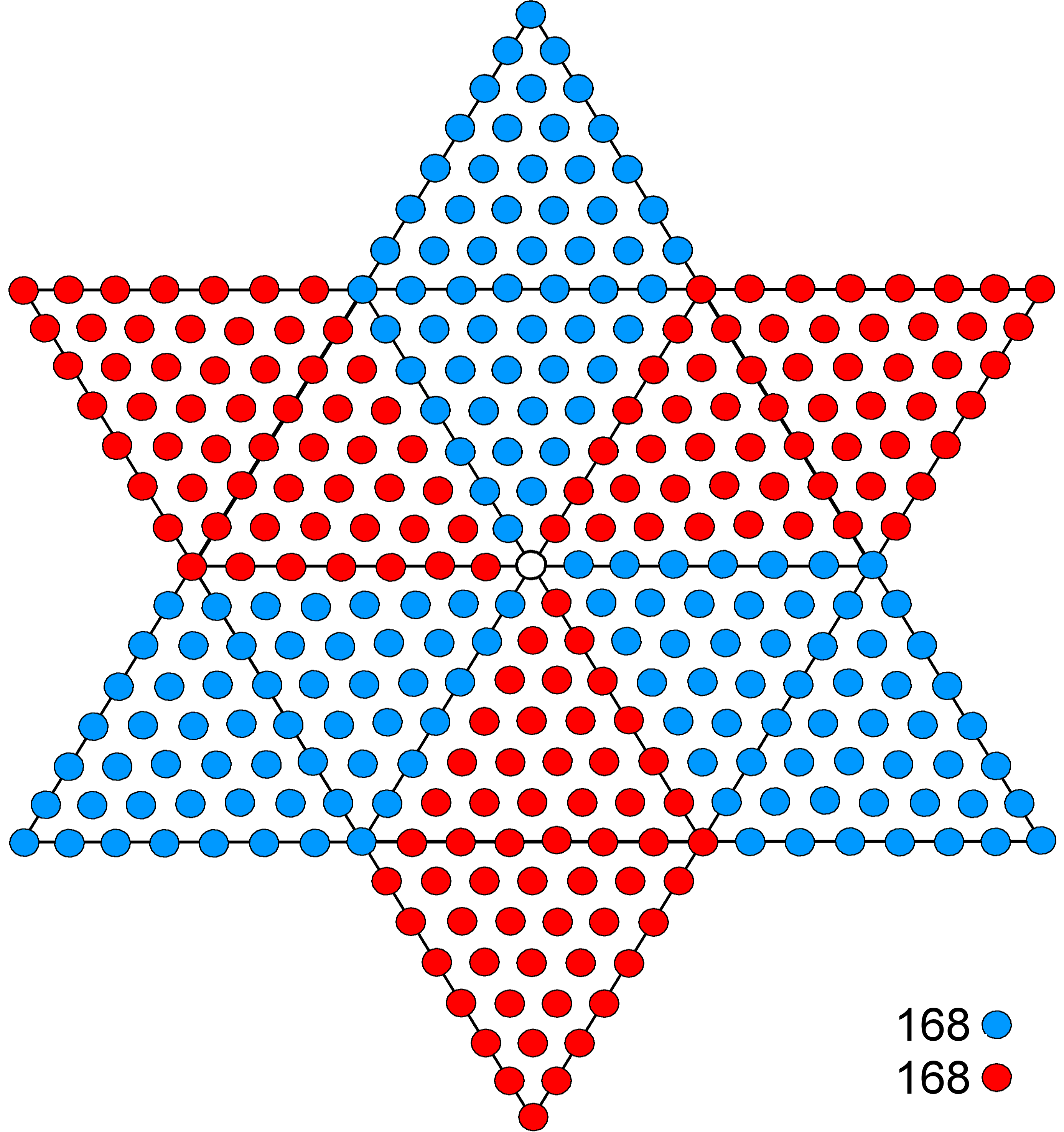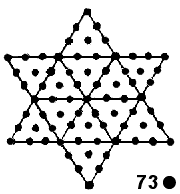<< Previous    1...   52  53  54  55      Next >>

#56 How the hexagram determines the superstring structural parameter 336

 336 =The 22nd triangular number is

1 + 2 + 3 +... + 22 = 11×23 = 253.

It is represented by an equilateral triangular array of 253 dots, with 22 dots along each side of the array. 252 dots surround its centre, where 252 is the sum of the first 21 integers after 1:

252 = 2 + 3 + 4 +... + 22.

The hexagram (or Star of David) that is formed by the intersection of two such arrays has six triangular points with eight dots arranged along each side. A point of the star has (1+2+3+4+5+6+7+8=36) dots. The complete star is composed of six parallelograms, with eight dots along each side. A separate parallelogram comprises (82=64) dots. When part of the star, it shares eight dots with its neighbour on one side and eight dots with its neighbour on the other side. It contributes (64-8=56) dots to the total population of dots surrounding the centre of the star, which is (56×6=336) dots. Each triangular array contributes (336/2=168) dots, so that the pair of intersecting triangles forming the hexagram expresses the split into two equal numbers:

336 = 168 + 168

that is characteristic of holistic systems embodying this parameter. In particular, each complete revolution about its axis of spin of a helical whorl of the UPA/E8×E8 heterotic superstring comprises 336 circular turns, of which 168 turns make up an outer half-revolution of the whorl and 168 turns comprise an inner half-revolution. This is the superstring manifestation of the pair of intersecting triangles in the hexagram, whose traditional meaning is one of symbolising the union of Divine Spirit and matter.

The superstring structural parameter 336 is the arithmetic mean of the squares of the first 31 integers:

336 = (12 + 22 + 32 +... + 312)/31,

where 31 is the number value of EL ("God"), which is the Godname assigned to Chesed, the first Sephirah of Construction.

When constructed from 1st-order tetractyses, the hexagram has 73 yods, where 73 is the number value of Chokmah:

 73 =The Archangel assigned to this Sephirah is Raziel, whose number value is 248. This number is the dimension of the rank-8, exceptional Lie group E8, whose symmetries belong to the unified force between E8×E8 heterotic superstrings. The UPA described by Besant & Leadbeater is their subquark state. The hexagram therefore provides a connection between the structural and dynamical parameters of this state of the E8×E8 heterotic superstring.

 << Previous    1...   52  53  54  55      Next >>

Home